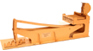The ternary calculating machine of Thomas Fowler home - about - contact Thomas Fowler Balanced ternary arithmetic Fowler's binary and ternary tables DeMorgan's description The reconstruction About the machine Photo gallery References and links Acknowledgements Wanted Fowler's Binary and Ternary TablesOne of Thomas Fowler’s many professions was as Treasurer of the Poor Law Union. This was an organization that collected fees (establishment charges) from each parish in the Union to aid the poor in the community. The amount of the fee was proportional to the value (assessment) of the parish. Thus, Fowler had to calculate the value of each parish relative to the Union as a whole, and then knowing the overall fees to be collected by the entire Union, calculate the fraction that each parish owes. This task was made much more complicated by the pre-decimal English currency (abbreviated as £sd after the symbols for pounds, shillings and pence). There were twenty shillings to a pound, twelve pence to a shilling and four farthings to a penny. When calculating proportions as Fowler needed to do, each monetary value would be converted into farthings before doing any calculations. With 960 farthings to the pound, these numbers became very large. The process was tedious, but decimal British currency was still over 130 years away. When faced with this huge task, Fowler developed a system which used lower bases to ease his calculating burden. In 1838, he published his Tables for Facilitating Arithmetical Calculations with the subtitle Intended for Calculating the Proportionate Charges on the Parishes in Poor Law Unions. This book contains a table of binary numbers for values from decimal 1 to 130048, and a table of balanced ternary numbers from decimal 1 to 3985807. Fowler also describes in detail how he uses these tables to simplify the particular type of calculations required for Poor Law Unions. Details of Fowler’s method are given here.A sample page of the ternary tables is shown at the left. For each decimal number in the leftmost column, the next two columns identify the powers of three, both positive and negative, to express that number in balanced ternary. The column at the far right is used to expand the range of the table by a factor of 3^6. Each number in the center of the block of three can be expressed in balanced ternary by adding six to the value of the indicies shown to the left. The number directly above and below indicate the range over which this value can be used. For example, numbers between 1094 and 3280 can be determined by converting the middle number, 2187, into balanced ternary, and then looking up the remainder in the leftmost columns of the table, keeping in mind that the remainder may actually be a negative value. Fowler’s publication of his book preceded his calculating machine by two years. The machine is clearly designed as a mechanical aid to this particular technique of calculation. Fowler’s technique has definite advantages for the repetitive calculations required at the Poor Law Union, but for less repetitive problems, the conversion to and from balanced ternary might be more difficult than calculating the problem directly in decimal.